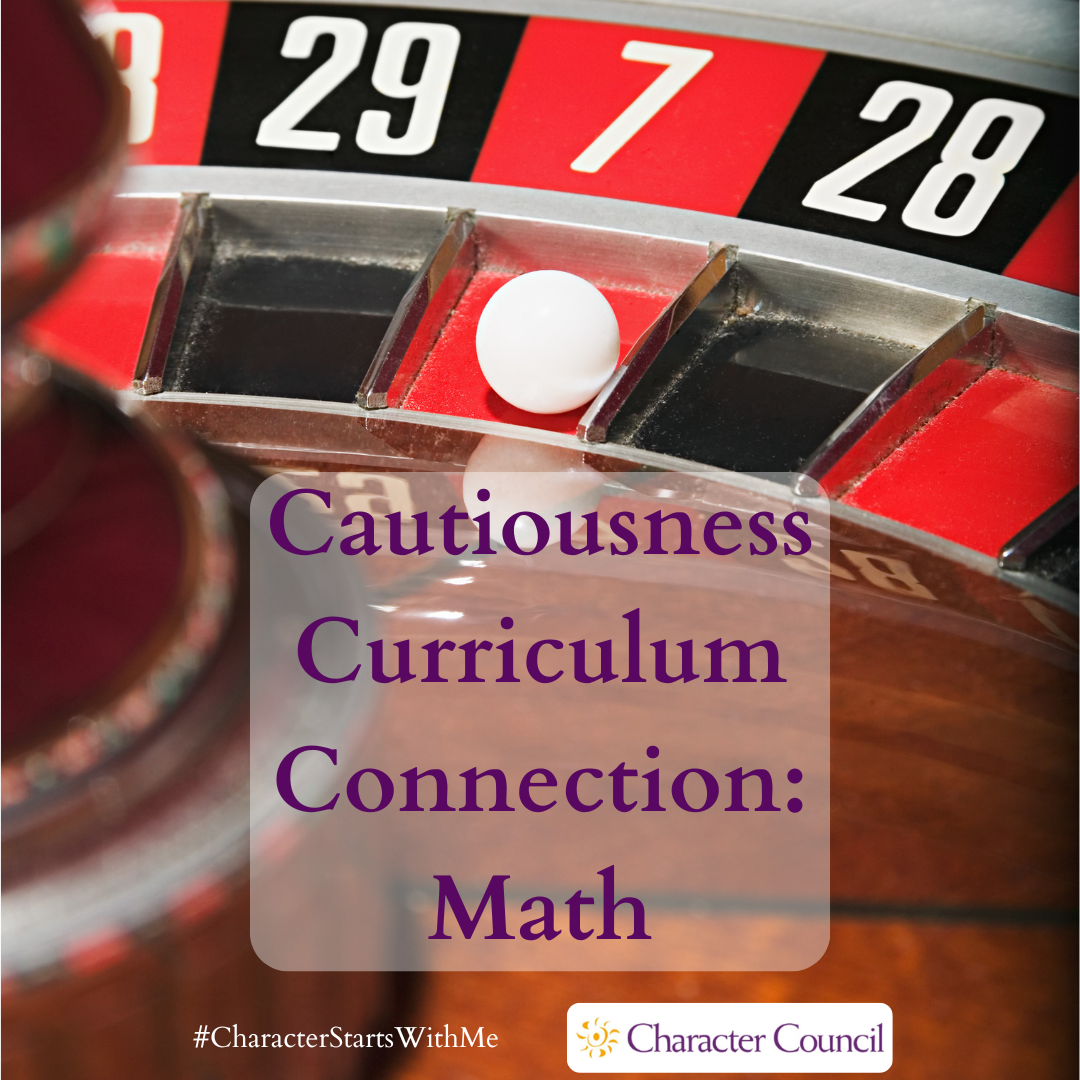Select Page# Cautiousness Curriculum Connection – Math

by | Jul 29, 2019 | Curriculum Connection | 0 commentsSerious gamblers know how to calculate the risk of any given decision is a game of chance. In deciding, you sometimes have to calculate the risk involved in that decision. A math lesson on probability and statistics can show you how to decide when all outcomes are known and calculatable. Discuss how to make decisions when that is not the case. Can you construct a logic model that may help you quantify a risk?